## 5.48. balance_path

Origin
Constraint

$\mathrm{𝚋𝚊𝚕𝚊𝚗𝚌𝚎}_\mathrm{𝚙𝚊𝚝𝚑}\left(\mathrm{𝙱𝙰𝙻𝙰𝙽𝙲𝙴},\mathrm{𝙽𝙾𝙳𝙴𝚂}\right)$

Arguments
 $\mathrm{𝙱𝙰𝙻𝙰𝙽𝙲𝙴}$ $\mathrm{𝚍𝚟𝚊𝚛}$ $\mathrm{𝙽𝙾𝙳𝙴𝚂}$ $\mathrm{𝚌𝚘𝚕𝚕𝚎𝚌𝚝𝚒𝚘𝚗}\left(\mathrm{𝚒𝚗𝚍𝚎𝚡}-\mathrm{𝚒𝚗𝚝},\mathrm{𝚜𝚞𝚌𝚌}-\mathrm{𝚍𝚟𝚊𝚛}\right)$
Restrictions
 $\mathrm{𝙱𝙰𝙻𝙰𝙽𝙲𝙴}\ge 0$ $\mathrm{𝙱𝙰𝙻𝙰𝙽𝙲𝙴}\le \mathrm{𝚖𝚊𝚡}\left(0,|\mathrm{𝙽𝙾𝙳𝙴𝚂}|-2\right)$ $\mathrm{𝚛𝚎𝚚𝚞𝚒𝚛𝚎𝚍}$$\left(\mathrm{𝙽𝙾𝙳𝙴𝚂},\left[\mathrm{𝚒𝚗𝚍𝚎𝚡},\mathrm{𝚜𝚞𝚌𝚌}\right]\right)$ $\mathrm{𝙽𝙾𝙳𝙴𝚂}.\mathrm{𝚒𝚗𝚍𝚎𝚡}\ge 1$ $\mathrm{𝙽𝙾𝙳𝙴𝚂}.\mathrm{𝚒𝚗𝚍𝚎𝚡}\le |\mathrm{𝙽𝙾𝙳𝙴𝚂}|$ $\mathrm{𝚍𝚒𝚜𝚝𝚒𝚗𝚌𝚝}$$\left(\mathrm{𝙽𝙾𝙳𝙴𝚂},\mathrm{𝚒𝚗𝚍𝚎𝚡}\right)$ $\mathrm{𝙽𝙾𝙳𝙴𝚂}.\mathrm{𝚜𝚞𝚌𝚌}\ge 1$ $\mathrm{𝙽𝙾𝙳𝙴𝚂}.\mathrm{𝚜𝚞𝚌𝚌}\le |\mathrm{𝙽𝙾𝙳𝙴𝚂}|$
Purpose

Consider a digraph $G$ described by the $\mathrm{𝙽𝙾𝙳𝙴𝚂}$ collection. Partition $G$ into a set of vertex disjoint paths in such a way that each vertex of $G$ belongs to a single path. $\mathrm{𝙱𝙰𝙻𝙰𝙽𝙲𝙴}$ is equal to the difference between the number of vertices of the largest path and the number of vertices of the smallest path.

Example
 $\left(\begin{array}{c}3,〈\begin{array}{cc}\mathrm{𝚒𝚗𝚍𝚎𝚡}-1\hfill & \mathrm{𝚜𝚞𝚌𝚌}-1,\hfill \\ \mathrm{𝚒𝚗𝚍𝚎𝚡}-2\hfill & \mathrm{𝚜𝚞𝚌𝚌}-3,\hfill \\ \mathrm{𝚒𝚗𝚍𝚎𝚡}-3\hfill & \mathrm{𝚜𝚞𝚌𝚌}-5,\hfill \\ \mathrm{𝚒𝚗𝚍𝚎𝚡}-4\hfill & \mathrm{𝚜𝚞𝚌𝚌}-4,\hfill \\ \mathrm{𝚒𝚗𝚍𝚎𝚡}-5\hfill & \mathrm{𝚜𝚞𝚌𝚌}-1,\hfill \\ \mathrm{𝚒𝚗𝚍𝚎𝚡}-6\hfill & \mathrm{𝚜𝚞𝚌𝚌}-6,\hfill \\ \mathrm{𝚒𝚗𝚍𝚎𝚡}-7\hfill & \mathrm{𝚜𝚞𝚌𝚌}-7,\hfill \\ \mathrm{𝚒𝚗𝚍𝚎𝚡}-8\hfill & \mathrm{𝚜𝚞𝚌𝚌}-6\hfill \end{array}〉\hfill \end{array}\right)$ $\left(\begin{array}{c}0,〈\begin{array}{cc}\mathrm{𝚒𝚗𝚍𝚎𝚡}-1\hfill & \mathrm{𝚜𝚞𝚌𝚌}-2,\hfill \\ \mathrm{𝚒𝚗𝚍𝚎𝚡}-2\hfill & \mathrm{𝚜𝚞𝚌𝚌}-3,\hfill \\ \mathrm{𝚒𝚗𝚍𝚎𝚡}-3\hfill & \mathrm{𝚜𝚞𝚌𝚌}-4,\hfill \\ \mathrm{𝚒𝚗𝚍𝚎𝚡}-4\hfill & \mathrm{𝚜𝚞𝚌𝚌}-4,\hfill \\ \mathrm{𝚒𝚗𝚍𝚎𝚡}-5\hfill & \mathrm{𝚜𝚞𝚌𝚌}-6,\hfill \\ \mathrm{𝚒𝚗𝚍𝚎𝚡}-6\hfill & \mathrm{𝚜𝚞𝚌𝚌}-7,\hfill \\ \mathrm{𝚒𝚗𝚍𝚎𝚡}-7\hfill & \mathrm{𝚜𝚞𝚌𝚌}-8,\hfill \\ \mathrm{𝚒𝚗𝚍𝚎𝚡}-8\hfill & \mathrm{𝚜𝚞𝚌𝚌}-8\hfill \end{array}〉\hfill \end{array}\right)$ $\left(\begin{array}{c}6,〈\begin{array}{cc}\mathrm{𝚒𝚗𝚍𝚎𝚡}-1\hfill & \mathrm{𝚜𝚞𝚌𝚌}-2,\hfill \\ \mathrm{𝚒𝚗𝚍𝚎𝚡}-2\hfill & \mathrm{𝚜𝚞𝚌𝚌}-3,\hfill \\ \mathrm{𝚒𝚗𝚍𝚎𝚡}-3\hfill & \mathrm{𝚜𝚞𝚌𝚌}-4,\hfill \\ \mathrm{𝚒𝚗𝚍𝚎𝚡}-4\hfill & \mathrm{𝚜𝚞𝚌𝚌}-5,\hfill \\ \mathrm{𝚒𝚗𝚍𝚎𝚡}-5\hfill & \mathrm{𝚜𝚞𝚌𝚌}-6,\hfill \\ \mathrm{𝚒𝚗𝚍𝚎𝚡}-6\hfill & \mathrm{𝚜𝚞𝚌𝚌}-7,\hfill \\ \mathrm{𝚒𝚗𝚍𝚎𝚡}-7\hfill & \mathrm{𝚜𝚞𝚌𝚌}-7,\hfill \\ \mathrm{𝚒𝚗𝚍𝚎𝚡}-8\hfill & \mathrm{𝚜𝚞𝚌𝚌}-8\hfill \end{array}〉\hfill \end{array}\right)$

In the first example we have the following four paths: $2\to 3\to 5\to 1$, $8\to 6$, 4, and 7. Since $\mathrm{𝙱𝙰𝙻𝙰𝙽𝙲𝙴}=3$ is the difference between the number of vertices of the largest path (i.e., 4) and the number of vertices of the smallest path (i.e., 1) the corresponding $\mathrm{𝚋𝚊𝚕𝚊𝚗𝚌𝚎}_\mathrm{𝚙𝚊𝚝𝚑}$ constraint holds.

All solutions

Figure 5.48.1 gives all solutions to the following non ground instance of the $\mathrm{𝚋𝚊𝚕𝚊𝚗𝚌𝚎}_\mathrm{𝚙𝚊𝚝𝚑}$ constraint: $\mathrm{𝙱𝙰𝙻𝙰𝙽𝙲𝙴}=0$, ${S}_{1}\in \left[1,2\right]$, ${S}_{2}\in \left[1,3\right]$, ${S}_{3}\in \left[3,5\right]$, ${S}_{4}\in \left[3,4\right]$, ${S}_{5}\in \left[2,5\right]$, ${S}_{6}\in \left[5,6\right]$, $\mathrm{𝚋𝚊𝚕𝚊𝚗𝚌𝚎}_\mathrm{𝚙𝚊𝚝𝚑}$$\left(\mathrm{𝙱𝙰𝙻𝙰𝙽𝙲𝙴},〈1{S}_{1},2{S}_{2},3{S}_{3},4{S}_{4},5{S}_{5},6{S}_{6}〉\right)$.

##### Figure 5.48.1. All solutions corresponding to the non ground example of the $\mathrm{𝚋𝚊𝚕𝚊𝚗𝚌𝚎}_\mathrm{𝚙𝚊𝚝𝚑}$ constraint of the All solutions slot; the $\mathrm{𝚒𝚗𝚍𝚎𝚡}$ attribute is displayed as indices of the $\mathrm{𝚜𝚞𝚌𝚌}$ attribute and all vertices of a same path are coloured by the same colour.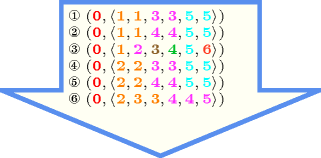Typical
$|\mathrm{𝙽𝙾𝙳𝙴𝚂}|>2$
Symmetry

Items of $\mathrm{𝙽𝙾𝙳𝙴𝚂}$ are permutable.

Arg. properties

Functional dependency: $\mathrm{𝙱𝙰𝙻𝙰𝙽𝙲𝙴}$ determined by $\mathrm{𝙽𝙾𝙳𝙴𝚂}$.

Counting
 Length ($n$) 2 3 4 5 6 7 8 Solutions 3 13 73 501 4051 37633 394353

Number of solutions for $\mathrm{𝚋𝚊𝚕𝚊𝚗𝚌𝚎}_\mathrm{𝚙𝚊𝚝𝚑}$: domains $0..n$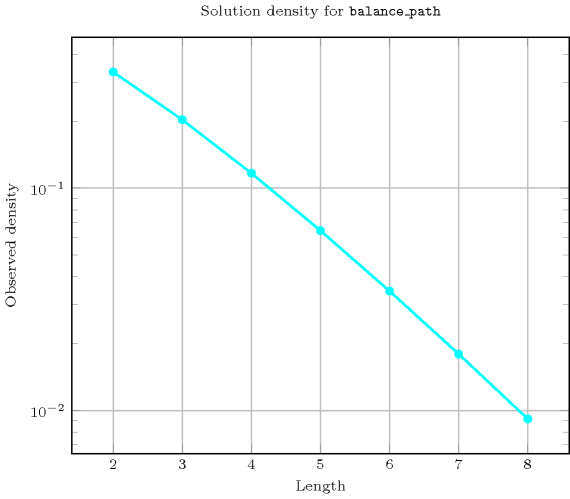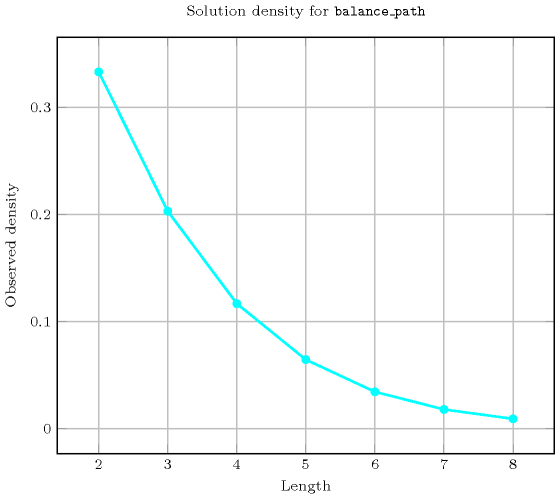Length ($n$)2345678
Total31373501405137633394353
 Parameter value

037371211201504162161
1-612200210886224416
2--24601560525097776
3---1203601092062160
4----720252087360
5-----504020160
6------40320

Solution count for $\mathrm{𝚋𝚊𝚕𝚊𝚗𝚌𝚎}_\mathrm{𝚙𝚊𝚝𝚑}$: domains $0..n$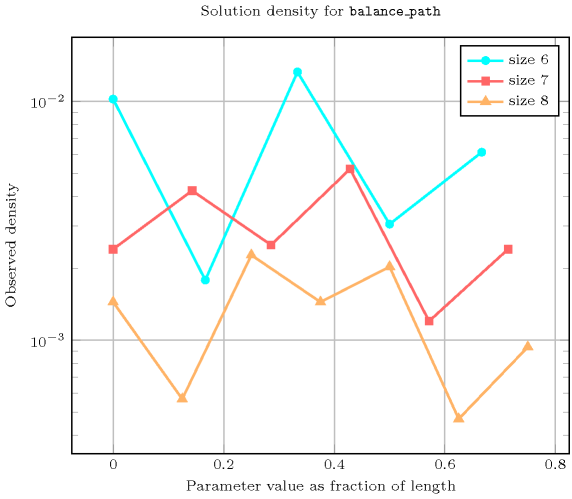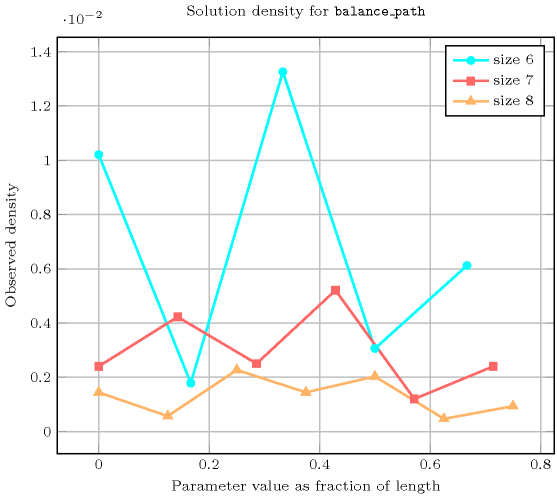related: $\mathrm{𝚋𝚊𝚕𝚊𝚗𝚌𝚎}$ (equivalence classes correspond to vertices in same path rather than variables assigned to the same value), $\mathrm{𝚙𝚊𝚝𝚑}$ (do not care how many paths but how balanced the paths are).

Keywords
Arc input(s)

$\mathrm{𝙽𝙾𝙳𝙴𝚂}$

Arc generator
$\mathrm{𝐶𝐿𝐼𝑄𝑈𝐸}$$↦\mathrm{𝚌𝚘𝚕𝚕𝚎𝚌𝚝𝚒𝚘𝚗}\left(\mathrm{𝚗𝚘𝚍𝚎𝚜}\mathtt{1},\mathrm{𝚗𝚘𝚍𝚎𝚜}\mathtt{2}\right)$

Arc arity
Arc constraint(s)
$\mathrm{𝚗𝚘𝚍𝚎𝚜}\mathtt{1}.\mathrm{𝚜𝚞𝚌𝚌}=\mathrm{𝚗𝚘𝚍𝚎𝚜}\mathtt{2}.\mathrm{𝚒𝚗𝚍𝚎𝚡}$
Graph property(ies)
 $•$$\mathrm{𝐌𝐀𝐗}_\mathrm{𝐍𝐒𝐂𝐂}$$\le 1$ $•$$\mathrm{𝐌𝐀𝐗}_\mathrm{𝐈𝐃}$$\le 1$ $•$$\mathrm{𝐑𝐀𝐍𝐆𝐄}_\mathrm{𝐍𝐂𝐂}$$=\mathrm{𝙱𝙰𝙻𝙰𝙽𝙲𝙴}$

Graph class
$\mathrm{𝙾𝙽𝙴}_\mathrm{𝚂𝚄𝙲𝙲}$

Graph model

In order to express the binary constraint that links two vertices one has to make explicit the identifier of the vertices. This is why the $\mathrm{𝚋𝚊𝚕𝚊𝚗𝚌𝚎}_\mathrm{𝚙𝚊𝚝𝚑}$ constraint considers objects that have two attributes:

• One fixed attribute $\mathrm{𝚒𝚗𝚍𝚎𝚡}$ that is the identifier of the vertex,

• One variable attribute $\mathrm{𝚜𝚞𝚌𝚌}$ that is the successor of the vertex.

We use the graph property $\mathrm{𝐌𝐀𝐗}_\mathrm{𝐍𝐒𝐂𝐂}$$\le 1$ in order to specify the fact that the size of the largest strongly connected component should not exceed one. In fact each root of a tree is a strongly connected component with a single vertex. The graph property $\mathrm{𝐌𝐀𝐗}_\mathrm{𝐈𝐃}$$\le 1$ constraints the maximum in-degree of the final graph to not exceed 1. $\mathrm{𝐌𝐀𝐗}_\mathrm{𝐈𝐃}$ does not consider loops: This is why we do not have any problem with the final node of each path.

Parts (A) and (B) of Figure 5.48.2 respectively show the initial and final graph associated with the first example of the Example slot. Since we use the $\mathrm{𝐑𝐀𝐍𝐆𝐄}_\mathrm{𝐍𝐂𝐂}$ graph property, we show the connected components of the final graph. The constraint holds since all the vertices belong to a path and since $\mathrm{𝙱𝙰𝙻𝙰𝙽𝙲𝙴}$ $=$ $\mathrm{𝐑𝐀𝐍𝐆𝐄}_\mathrm{𝐍𝐂𝐂}$ $=$ 3.

##### Figure 5.48.2. Initial and final graph of the $\mathrm{𝚋𝚊𝚕𝚊𝚗𝚌𝚎}_\mathrm{𝚙𝚊𝚝𝚑}$ constraint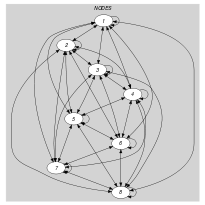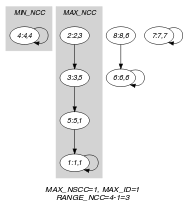(a) (b)